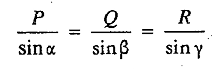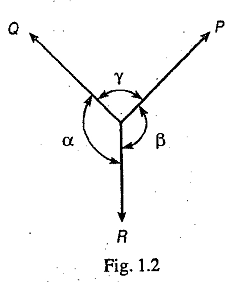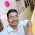### Lami's Theorem

Lami's Theorem states that if three coplaner forces acting at a point be in equilibrium, then each force is proportional to the sine of the angle between the other two forces. Mathematicallywhere P, Q and R are the three forces and α, β and γ are the angles as shown in Fig 1.2.

1.1.2.